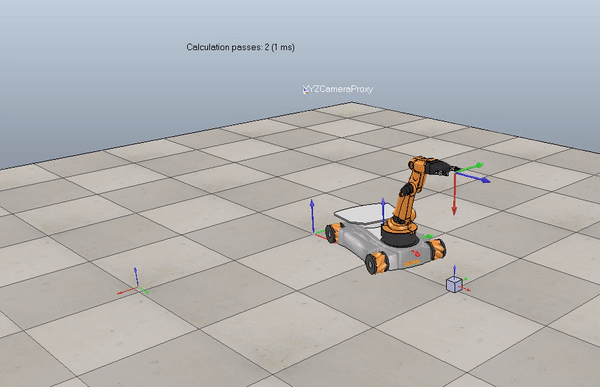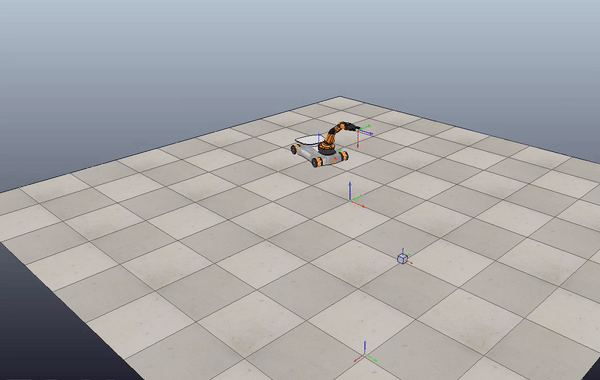## Sir Mix-A-Bot### Project information

The purpose of this project was to demonstrate understanding of the math behind robotic manipulation. I wrote Python code that plans a trajectory, calculates odometry, and performs feedback control. The code outputs a csv file, that can be imported into CoppeliaSim to visualize results.

After generating a reference trajectory, I worked towards simulating the YouBot's kinematics. I wrote a function, NextState, that inputs the current robot configuration and joint speeds. The function uses first-order Euler integration to calculate wheel and joint angles at the next time step. I then calculated the chasis configuration based on the change in wheel angles over the time step. To do this, I started with the body twist of a four-mecanum-wheel robot. Using matrix exponentials I determined the transformation from the space frame to the robot chasis. Combining these configuration variables gives the entire YouBot configuration at the next time step.